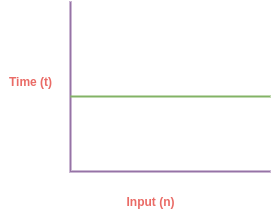# Part-3: Constant Time O(1) Complexity

## What is Constant Time O(1) Complexity?

Whan an algorithm runs independent of it's input size (n), execution time remains the same no matter how many time you run the same algorithm.In last tutorial we learned how to calculate time/space complexity of given algorithm. We learn constant time complexity by some examples:

```function sum() {
return 1 + 2; // 1 time unit
}

// time complexity
T(n) = 1 = O(1)

// a and b are constant for each execution
// input is not growing during execution
// no matter how many time you run code below
// execution time remain constant
function sum(a, b) {
return a + b; // 1 time unit
}

// time complexity
T(n) = 1 = O(1)```

In above code, no matter how many time we execute above functions their execution time will remain constant. During execution input size is not growing and always remain constant.

Therefore, time complexity of above functions is O(1). In big O notation we always care about bigger term from the equation.

## How to find out time/space complexity of Constant Time O(1) complexity?

You can use following steps in order to figure out time/space complexity of any algorithm:

• Calculate complexity of each statement
• Add up all the statements
• Take the bigger terms from the equation

Using above steps you can determine complexity of any algorithm. Let's look at some more examples of Constant Time O(1).

```// Problem: algorithm for even/odd numbers
function evenOrOdd(n) {
return (n%2 === 0) ? 'event' : 'odd; // 1 unit of time
}

T(n) = 1 = O(1)

// Assigning value to variable
const a = 1; // O(1)

// Defining an array
const arr = [1, 2, 3]; // O(1)

// Assigning new value to array
arr = 12; // O(1)

// Accessing array value
console.log(arr); // O(1)

// Arry push and pop operation
arr.pop();   // O(1)
arr.push(2); // O(1)```

In next tutorial, we will learn about Linear time complexity O(n).